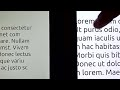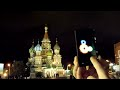Jogl/JogAmp on the Web, Desktop and Mobile 2012 Presenting JogAmp's upcoming release on PC and mobile devices, for Web applications and stand-alone. Running on desktop PCs (x86_32 and x86_64) Linux, Windows, OSX, Solaris - as well as on...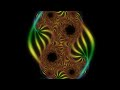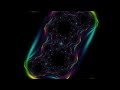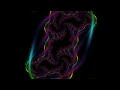OpenGL_FractalBitmapOrbitTrappig_Julia_Transform_T23_JOGL Another quick fragment shader variation that calculates a julia-set fractal, but instead of coloring the fragments using the iteration count of the IFS, the shader analizes the sequence of...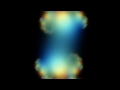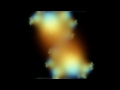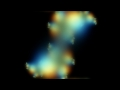OpenGL_FractalBitmapOrbitTrappig_Julia_Transform_T22_JOGL Another quick fragment shader variation that calculates a julia-set fractal, but instead of coloring the fragments using the iteration count of the IFS, the shader analizes the sequence of...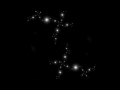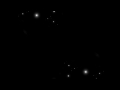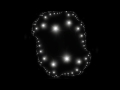OpenGL_FractalBitmapOrbitTrappig_Julia_Transform_T21_JOGL Another quick fragment shader variation that calculates a julia-set fractal, but instead of coloring the fragments using the iteration count of the IFS, the shader analizes the sequence of...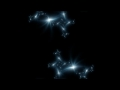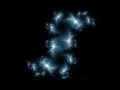OpenGL_FractalBitmapOrbitTrappig_Julia_Transform_T20_JOGL Another quick fragment shader variation that calculates a julia-set fractal, but instead of coloring the fragments using the iteration count of the IFS, the shader analizes the sequence of...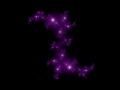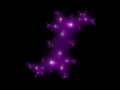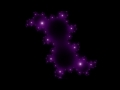OpenGL_FractalBitmapOrbitTrappig_Julia_Transform_T19_JOGL Another quick fragment shader variation that calculates a julia-set fractal, but instead of coloring the fragments using the iteration count of the IFS, the shader analizes the sequence of...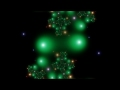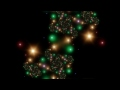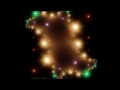OpenGL_FractalBitmapOrbitTrappig_Julia_Transform_T18_JOGL sigh* Flares again ... another quick fragment shader variation that calculates a julia-set fractal, but instead of coloring the fragments using the iteration count of the IFS, the shader analizes...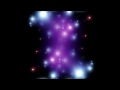OpenGL_FractalBitmapOrbitTrappig_Julia_Transform_T17_JOGL Again another quick fragment shader variation that calculates a julia-set fractal, but instead of coloring the fragments using the iteration count of the IFS, the shader analizes the sequence...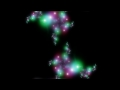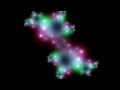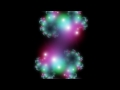OpenGL_FractalBitmapOrbitTrappig_Julia_Transform_T16_JOGL Those flares again :) Another quick fragment shader variation that calculates a julia-set fractal, but instead of coloring the fragments using the iteration count of the IFS, the shader analizes...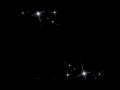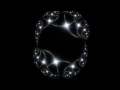OpenGL_FractalBitmapOrbitTrappig_Julia_Transform_T15_JOGL Those flares are driving me nuts :) Again another quick fragment shader variation that calculates a julia-set fractal, but instead of coloring the fragments using the iteration count of the...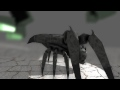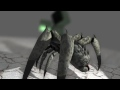OpenGL Skeletal Animation using JOGL 2.0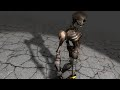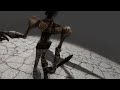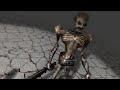MD2 Keyframe Animation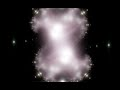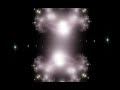OpenGL_FractalBitmapOrbitTrappig_Julia_Transform_T14_JOGL Another quick fragment shader variation that calculates a julia-set fractal, but instead of coloring the fragments using the iteration count of the IFS, the shader analizes the sequence of...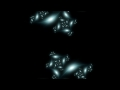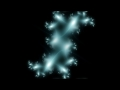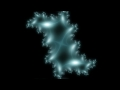OpenGL_FractalBitmapOrbitTrappig_Julia_Transform_T09_JOGL And yet another fragment shader variation that calculates a julia-set fractal, but instead of coloring the fragments using the iteration count of the IFS, the shader analizes the sequence of...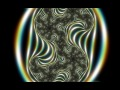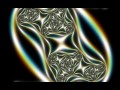OpenGL_FractalBitmapOrbitTrappig_Julia_Transform_T03_JOGL Yet another fragment shader variation that calculates a julia-set fractal, but instead of coloring the fragments using the iteration count of the IFS, the shader analizes the sequence of points...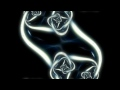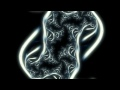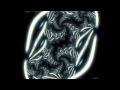OpenGL_FractalBitmapOrbitTrappig_Julia_Transform_T01_JOGL Fragment shader that calculates a julia-set fractal, but instead of coloring the fragments using the iteration count of the IFS, the shader analizes the sequence of points that was generated...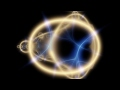OpenGL_FractalBitmapOrbitTrappig_Mandelbrot_Overview_T01-T39_JOGL An overview of nearly ~40 variations of my fragment shader that calculates a mandelbot fractal, but instead of coloring the fragments using the iteration count of the IFS, the shader analizes...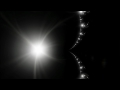OpenGL_FractalBitmapOrbitTrappig_Mandelbrot_ZoomIn_T22_JOGL And again another variation of my fragment shader that calculates a mandelbot fractal, but instead of coloring the fragments using the iteration count of the IFS, the shader analizes the sequence...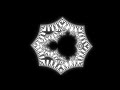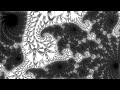OpenGL_FractalBitmapOrbitTrappig_Mandelbrot_ZoomIn_T28_JOGL Again another variation of my fragment shader that calculates a mandelbot fractal, but instead of coloring the fragments using the iteration count of the IFS, the shader analizes the sequence...OpenGL_FractalBitmapOrbitTrappig_Mandelbrot_ZoomIn_T27_JOGL Yet another variation of my fragment shader that calculates a mandelbot fractal, but instead of coloring the fragments using the iteration count of the IFS, the shader analizes the sequence...

 « Previous Page | Page (2 of 8) | Next Page »